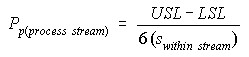# pp (stream)

## Assessing Performance as if Process Stream Differences were Removed

If the process under study contains multiple lines, tools, or stations (process streams), the losses from differences between these process streams should be understood. pp(process stream) is a measure of the process performance within process streams. If no process stream differences exist, and the process is on target, ppm would be equal to pp(process stream). pp(process stream) is calculated bywhere s(within stream) is the square-root of the average of the variances within each process stream calculated bywhere J is the number of process streams.

Another useful diagnostic measure related to this is the % Process Stream Difference.